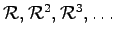# 2. Discrete Problems and Discretization

Methods designed to transform continuous mathematical problems - in most cases partial differential equations - into algebraic problems are called discretization methods. Such a continuous problem is usually given by a model for the governing physical processes which are determined by the physical reality to be investigated. The problem is given on a continuous space which is usually a subset of. This means that one is faced with at least an ordinary differential equation (ODE) or a partial differential equation (PDE) that optionally contains temporal derivatives.

Discretization considers the fact that analytical methods which are known from functional analysis cannot be directly applied to functions stored in a computer, and an assumption on the functional structure of the single elements has to be made.

One main aspect of this chapter is the development of a formalism which is designed for writing expressions in the context of discretization. It is shown that such a formalism can be used for a concise definition of the topological as well as the functional structure of the respective discretization formulae. This formalism can be used for the specification of computer programs, when such a formalism contains sufficient information to carry out all operations which are required for the evaluation of the formulae.